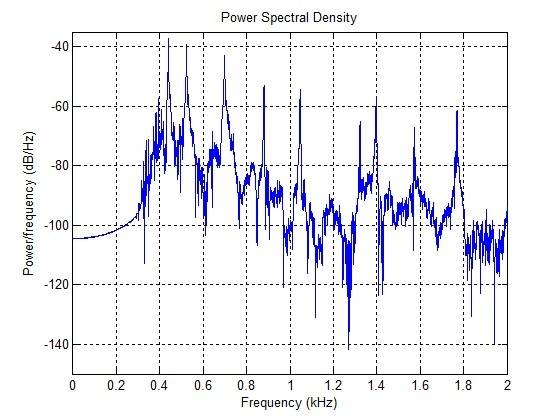# Identifying Fundamental Frequencies

## Homework Statement

I have made a plot of a given .wav file in Matlab. The problem asks: find the fundamental frequencies of the 3 vibrating strings, and identify some of their harmonics.

## Homework Equations

3. The Attempt at a Solution [/B]

So I made a Matlab plot of the power spectrum over the range 0 to 2000 Hz (the range we are required to plot):So how can I identify the fundamental frequencies using this plot (or by any other method)? And what would be their "harmonics"?

Any explanation would be greatly appreciated.

Orodruin
Staff Emeritus
Homework Helper
Gold Member
2021 Award
How would you approach the problem if there was one vibrating string? What is charachteristic for the fundamental frequency of a string?

How would you approach the problem if there was one vibrating string? What is charachteristic for the fundamental frequency of a string?

That is exactly my question. How would you approach this problem? I was told that the vibrating strings should have fundamental frequencies of around 440, 523 and 698 Hz. But how can we see that from the graph?

P. S. the .wav file I am plotting is a digitized recording of a three note chord being played on a piano.

Orodruin
Staff Emeritus
Homework Helper
Gold Member
2021 Award
Did you actually look at the graph and try to identify the resonant frequencies?

•roam
Thank you, yes the first resonance peak is at 440 Hz, the second one is at 523 Hz, and the third one is at 698 Hz.

There is also a fourth peak in the graph at ##881 \ Hz##. Is it valid to say that this is the second harmonic of the fundamental frequency ##439.8 \ Hz,## since ##881/2 \approx 440##?

I also identified a few other overtones of the 3 fundamental frequencies, but they are only approximately related to the fundamental frequency by a factor of N (= integer).

Orodruin
Staff Emeritus
Homework Helper
Gold Member
2021 Award
Thank you, yes the first resonance peak is at 440 Hz, the second one is at 523 Hz, and the third one is at 698 Hz.

There is also a fourth peak in the graph at ##881 \ Hz##. Is it valid to say that this is the second harmonic of the fundamental frequency ##439.8 \ Hz,## since ##881/2 \approx 440##?

Yes, this is where you expect the first overtone to be. In general, the overtones for an oscillating string are expected to be located at integer multiples of the fundamental frequency.

I also identified a few other overtones of the 3 fundamental frequencies, but they are only approximately related to the fundamental frequency by a factor of N (= integer).
Just looking at the diagram, I would say that the correspondence to integer multiples of the fundamental frequencies is pretty good. There are many factors which could result in not getting the exact corresponding values, including rounding errors, non-ideal strings, etc.

I would say every single peak in the diagram can be directly related to one of the fundamental frequencies.

•roam
Thank you so much for the explanation, it makes perfect sense now.

Merlin3189
Homework Helper
Gold Member
•